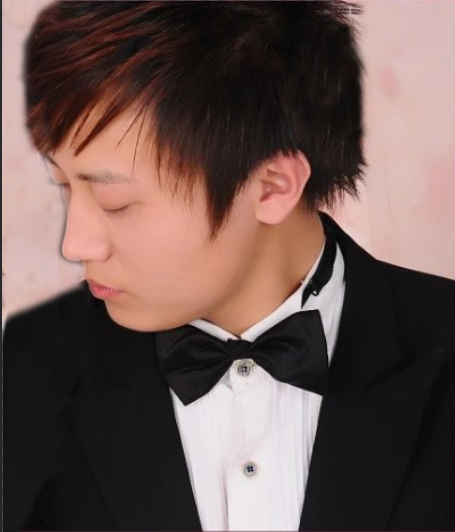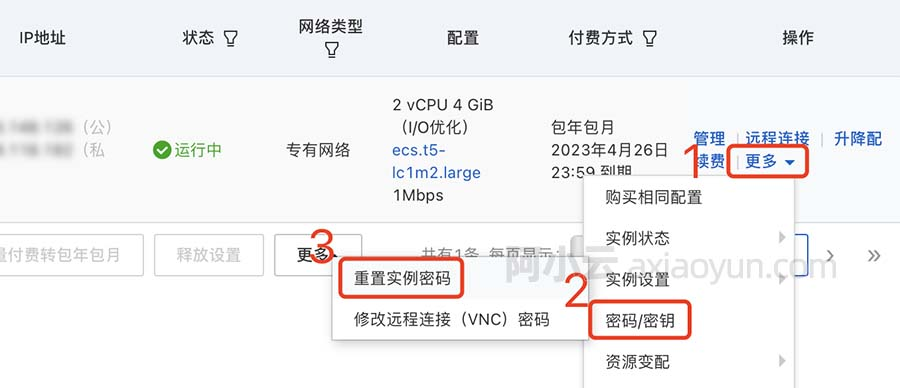字符串的全排列和组合算法(转)

+关注继续查看

1. #include<iostream>
2. using namespace std;
3. #include<assert.h>
4.
5. void Permutation(char* pStr, char* pBegin)
6. {
7.     assert(pStr && pBegin);
8.
9.     if(*pBegin == '\0')
10.         printf("%s\n",pStr);
11.     else
12.     {
13.         for(char* pCh = pBegin; *pCh != '\0'; pCh++)
14.         {
15.             swap(*pBegin,*pCh);
16.             Permutation(pStr, pBegin+1);
17.             swap(*pBegin,*pCh);
18.         }
19.     }
20. }
21.
22. int main(void)
23. {
24.     char str[] = "abc";
25.     Permutation(str,str);
26.     return 0;
27. }

1. //k表示当前选取到第几个数，m表示共有多少个数
2. void Permutation(char* pStr,int k,int m)
3. {
4.     assert(pStr);
5.
6.     if(k == m)
7.     {
8.         static int num = 1;  //局部静态变量，用来统计全排列的个数
9.         printf("第%d个排列\t%s\n",num++,pStr);
10.     }
11.     else
12.     {
13.         for(int i = k; i <= m; i++)
14.         {
15.             swap(*(pStr+k),*(pStr+i));
16.             Permutation(pStr, k + 1 , m);
17.             swap(*(pStr+k),*(pStr+i));
18.         }
19.     }
20. }
21.
22. int main(void)
23. {
24.     char str[] = "abc";
25.     Permutation(str , 0 , strlen(str)-1);
26.     return 0;
27. }

1. #include<iostream>
2. using namespace std;
3. #include<assert.h>
4.
5. //在[nBegin,nEnd)区间中是否有字符与下标为pEnd的字符相等
6. bool IsSwap(char* pBegin , char* pEnd)
7. {
8.     char *p;
9.     for(p = pBegin ; p < pEnd ; p++)
10.     {
11.         if(*p == *pEnd)
12.             return false;
13.     }
14.     return true;
15. }
16. void Permutation(char* pStr , char *pBegin)
17. {
18.     assert(pStr);
19.
20.     if(*pBegin == '\0')
21.     {
22.         static int num = 1;  //局部静态变量，用来统计全排列的个数
23.         printf("第%d个排列\t%s\n",num++,pStr);
24.     }
25.     else
26.     {
27.         for(char *pCh = pBegin; *pCh != '\0'; pCh++)   //第pBegin个数分别与它后面的数字交换就能得到新的排列
28.         {
29.             if(IsSwap(pBegin , pCh))
30.             {
31.                 swap(*pBegin , *pCh);
32.                 Permutation(pStr , pBegin + 1);
33.                 swap(*pBegin , *pCh);
34.             }
35.         }
36.     }
37. }
38.
39. int main(void)
40. {
41.     char str[] = "baa";
42.     Permutation(str , str);
43.     return 0;
44. }

OK，到现在我们已经能熟练写出递归的方法了，并且考虑了字符串中的重复数据可能引发的重复数列问题。那么如何使用非递归的方法来得到全排列了？

1. #include<iostream>
2. #include<algorithm>
3. #include<cstring>
4. using namespace std;
5. #include<assert.h>
6.
7. //反转区间
8. void Reverse(char* pBegin , char* pEnd)
9. {
10.     while(pBegin < pEnd)
11.         swap(*pBegin++ , *pEnd--);
12. }
13. //下一个排列
14. bool Next_permutation(char a[])
15. {
16.     assert(a);
17.     char *p , *q , *pFind;
18.     char *pEnd = a + strlen(a) - 1;
19.     if(a == pEnd)
20.         return false;
21.     p = pEnd;
22.     while(p != a)
23.     {
24.         q = p;
25.         p--;
26.         if(*p < *q)  //找降序的相邻2数,前一个数即替换数
27.         {
28.              //从后向前找比替换点大的第一个数
29.             pFind = pEnd;
30.             while(*pFind < *p)
31.                 --pFind;
32.             swap(*p , *pFind);
33.             //替换点后的数全部反转
34.             Reverse(q , pEnd);
35.             return true;
36.         }
37.     }
38.     Reverse(a , pEnd);   //如果没有下一个排列,全部反转后返回false
39.     return false;
40. }
41.
42. int cmp(const void *a,const void *b)
43. {
44.     return int(*(char *)a - *(char *)b);
45. }
46. int main(void)
47. {
48.     char str[] = "bac";
49.     int num = 1;
50.     qsort(str , strlen(str),sizeof(char),cmp);
51.     do
52.     {
53.         printf("第%d个排列\t%s\n",num++,str);
54.     }while(Next_permutation(str));
55.     return 0;
56. }

1、全排列就是从第一个数字起每个数分别与它后面的数字交换。
2、去重的全排列就是从第一个数字起每个数分别与它后面非重复出现的数字交换。
3、全排列的非递归就是由后向前找替换数和替换点，然后由后向前找第一个比替换数大的数与替换数交换，最后颠倒替换点后的所有数据。

1. #include<iostream>
2. #include<vector>
3. #include<cstring>
4. using namespace std;
5. #include<assert.h>
6.
7. void Combination(char *string ,int number,vector<char> &result);
8.
9. void Combination(char *string)
10. {
11.     assert(string != NULL);
12.     vector<char> result;
13.     int i , length = strlen(string);
14.     for(i = 1 ; i <= length ; ++i)
15.         Combination(string , i ,result);
16. }
17.
18. void Combination(char *string ,int number , vector<char> &result)
19. {
20.     assert(string != NULL);
21.     if(number == 0)
22.     {
23.         static int num = 1;
24.         printf("第%d个组合\t",num++);
25.
26.         vector<char>::iterator iter = result.begin();
27.         for( ; iter != result.end() ; ++iter)
28.             printf("%c",*iter);
29.         printf("\n");
30.         return ;
31.     }
32.     if(*string == '\0')
33.         return ;
34.     result.push_back(*string);
35.     Combination(string + 1 , number - 1 , result);
36.     result.pop_back();
37.     Combination(string + 1 , number , result);
38. }
39.
40. int main(void)
41. {
42.     char str[] = "abc";
43.     Combination(str);
44.     return 0;
45. }

1. #include<iostream>
2. using namespace std;
3.
4. int a[] = {1,3,5,4,6};
5. char str[] = "abcde";
6.
7. void print_subset(int n , int s)
8. {
9.     printf("{");
10.     for(int i = 0 ; i < n ; ++i)
11.     {
12.         if( s&(1<<i) )         // 判断s的二进制中哪些位为1，即代表取某一位
13.             printf("%c ",str[i]);   //或者a[i]
14.     }
15.     printf("}\n");
16. }
17.
18. void subset(int n)
19. {
20.     for(int i= 0 ; i < (1<<n) ; ++i)
21.     {
22.         print_subset(n,i);
23.     }
24. }
25.
26.
27.
28. int main(void)
29. {
30.     subset(5);
31.     return 0;
32. }

题目：在8×8的国际象棋上摆放八个皇后，使其不能相互攻击，即任意两个皇后不得处在同一行、同一列或者同一对角斜线上。下图中的每个黑色格子表示一个皇后，这就是一种符合条件的摆放方法。请求出总共有多少种摆法。这就是有名的八皇后问题。解决这个问题通常需要用递归，而递归对编程能力的要求比较高。因此有不少面试官青睐这个题目，用来考察应聘者的分析复杂问题的能力以及编程的能力。

1. #include<iostream>
2. using namespace std;
3.
4. int g_number = 0;
5. void Permutation(int * , int  , int );
6. void Print(int * , int );
7.
8. void EightQueen( )
9. {
10.     const int queens = 8;
11.     int ColumnIndex[queens];
12.     for(int i = 0 ; i < queens ; ++i)
13.         ColumnIndex[i] = i;    //初始化
14.     Permutation(ColumnIndex , queens , 0);
15. }
16.
17. bool Check(int ColumnIndex[] , int length)
18. {
19.     int i,j;
20.     for(i = 0 ; i < length; ++i)
21.     {
22.         for(j = i + 1 ; j < length; ++j)
23.         {
24.             if( i - j == ColumnIndex[i] - ColumnIndex[j] || j - i == ColumnIndex[i] - ColumnIndex[j])   //在正、副对角线上
25.                 return false;
26.         }
27.     }
28.     return true;
29. }
30. void Permutation(int ColumnIndex[] , int length , int index)
31. {
32.     if(index == length)
33.     {
34.         if( Check(ColumnIndex , length) )   //检测棋盘当前的状态是否合法
35.         {
36.             ++g_number;
37.             Print(ColumnIndex , length);
38.         }
39.     }
40.     else
41.     {
42.         for(int i = index ; i < length; ++i)   //全排列
43.         {
44.             swap(ColumnIndex[index] , ColumnIndex[i]);
45.             Permutation(ColumnIndex , length , index + 1);
46.             swap(ColumnIndex[index] , ColumnIndex[i]);
47.         }
48.     }
49. }
50.
51. void Print(int ColumnIndex[] , int length)
52. {
53.     printf("%d\n",g_number);
54.     for(int i = 0 ; i < length; ++i)
55.         printf("%d ",ColumnIndex[i]);
56.     printf("\n");
57. }
58.
59. int main(void)
60. {
61.     EightQueen();
62.     return 0;
63. }

1. #include <iostream>
2. #include <list>
3. using namespace std;
4. list<int> list1;
5. void find_factor(int sum,int n)
6. {
7.     //递归出口
8.     if(n<=0||sum<=0)
9.         return;
10.     //输出找到的数
11.     if(sum==n)
12.     {
13.         list1.reverse();
14.         for(list<int>::iterator iter=list1.begin();iter!=list1.end();iter++)
15.             cout<<*iter<<"+";
16.         cout<<n<<endl;
17.         list1.reverse();
18.     }
19.     list1.push_front(n);
20.     find_factor(sum-n,n-1);//n放在里面
21.     list1.pop_front();
22.     find_factor(sum,n-1);//n不放在里面
23. }
24.
25. int main(void)
26. {
27.     int sum,n;
28.     cin>>sum>>n;
29.     cout<<"所有可能的序列，如下："<<endl;
30.     find_factor(sum,n);
31.     return 0;
32. }9497 01303 06336 051 Nod 1791 合法括号子段【分治+字符串】
1791 合法括号子段 基准时间限制：1 秒 空间限制：131072 KB 分值: 40 难度：4级算法题 有一个括号序列，现在要计算一下它有多少非空子段是合法括号序列。 合法括号序列的定义是： 1.空序列是合法括号序列。
847 011214 013186 0761 0C#语言之“中英文混合字符串对齐”的方法【转】

783 054292 06895 0

761

0

《2021云上架构与运维峰会演讲合集》

《零基础CSS入门教程》

《零基础HTML入门教程》